Publication date: 05/24/2021

## Multivariate Tests

In the following, E is the residual cross product matrix and H is the model cross product matrix. Diagonal elements of E are the residual sums of squares for each variable. Diagonal elements of H are the sums of squares for the model for each variable. In the discriminant analysis literature, E is often called W, where W stands for within.

Test statistics in the multivariate results tables are functions of the eigenvalues λ of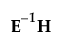. The following list describes the computation of each test statistic.

Note: After specification of a response design, the initial E and H matrices are premultiplied by M and postmultiplied by M.

Wilks’ Lambda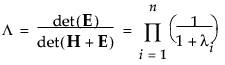Pillai’s Trace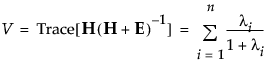Hotelling-Lawley Trace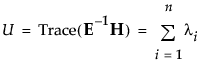Roy’s Max Root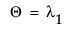, the maximum eigenvalue of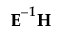.

E and H are defined as follows: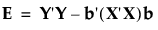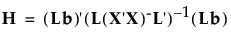where b is the estimated vector for the model coefficients and A- denotes the generalized inverse of a matrix A.

The whole model L is a column of zeros (for the intercept) concatenated with an identity matrix having the number of rows and columns equal to the number of parameters in the model. L matrices for effects are subsets of rows from the whole model L matrix.